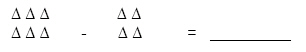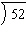Name:    3rd Grade Basic Math Test 2

Multiple Choice
Identify the choice that best completes the statement or answers the question.

1.

Computation – Decimals – RIT 161 – 170

\$5.25

-  3.14
 a. \$1.21 d. \$2.39 b. \$2.11 e. \$4.31 c. \$8.39

2.

Computation – Whole Numbers – RIT 161 – 170a. 10 d. 4 b. 3 e. 5 c. 2

3.

63
+ 34
 a. 37 d. 98 b. 97 e. 31 c. 71

4.

26

- 5
 a. 21 d. 32 b. 11 e. 31 c. 20

5.

99
-56
 a. 34 d. 43 b. 155 e. 42 c. 53

6.

If Susan reads 3 more books, how many books will she read in all?
 a. 9 d. 6 b. 3 e. 8 c. 12

7.

834
-  22
 a. 816 d. 756 b. 856 e. 812 c. 712

8.

Computation – Decimals – RIT 171 – 180

6.24
+2.53
 a. 877 d. 87.7 b. 8.78 e. 8.77 c. 8.87

9.

Computation – Fractions – RIT 171 – 180
3/5 + 1/5 =
 a. 3/5 d. 4/5 b. 3/25 e. 2/5 c. 4/10

10.

Computation – Whole Numbers – RIT 171 – 180
Juan had 24 crayons. He lost 10 of them.
How many crayons does Juan have now?
 a. 34 d. 18 b. 23 e. 10 c. 14

11.

36
+ 8
 a. 45 d. 44 b. 32 e. 34 c. 28

12.

321
+ 49
 a. 360 d. 328 b. 368 e. 370 c. 378

13.

78
-39
 a. 39 d. 27 b. 41 e. 47 c. 31

14.Choose the number sentence below that matches the picture.
 a. 4 x 6 d. 24 + 4 b. 6 + 4 e. 24 – 6 c. 4 x 24

15.

7,456
+4,872
 a. 12,428 d. 141,418 b. 12,328 e. 12,412 c. 12,318

16.

23
x 3
 a. 56 d. 59 b. 66 e. 68 c. 69

17.

46

x 2
 a. 68 d. 92 b. 102 e. 101 c. 82

18.

7 + 2 = 2 + _____
 a. 5 d. 11 b. 9 e. 7 c. 2

19.a. 8 R4 d. 8 R3 b. 9 R2 e. 7 R10 c. 7 R4

20.

Round 68 to the nearest tens place.
 a. 78 d. 80 b. 70 e. 100 c. 60

21.

243
x 15
 a. 1,458 d. 3,635 b. 5,645 e. 3,545 c. 3,645